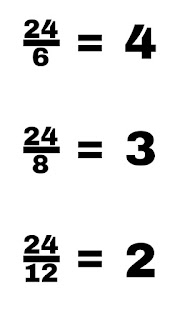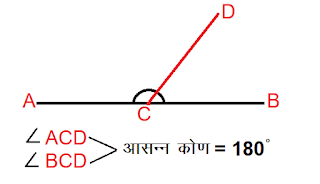## how to calculate lcm using prime factorization faster

### Factorization method

In this article we learn “how to calculate lcm using prime factorization“. Numbers are factorized to find the least common multiple by the factorization method. We will consider this method as set by set.

First Step – We do the prime factors of the given numbers.

Example – If we have to find the least common denominator of 7, 14, 24. So first we will find their prime factors.

• Prime prime factors of 8?

The prime factor of 8 is 2 × 2 × 2

• Prime prime factors of 16?

Prime factorization of 16 = 2 x 2 x 2 x 2

• Prime factorization of 24?

Prime factorization of 24 = 2x2x2x3

Second Step – In this step we organize the number. Lets keep small numbers first.

Prime factorization of 8   = 2 x2x2
Prime factorization of 16 = 2x2x2x2
Prime factorization of 24 = 2x2x2x3

Third Step – After arranging all the numbers the common numbers are chosen.

Prime factorization of 8   = 2 x2x2
Prime factorization of 16 = 2x2x2x2
Prime factorization of 24 = 2x2x2x3

Hence the common number is 2x2x2.

Fourth Step – After selecting common numbers, we include the remaining numbers.

Prime factorization of 8 = 2 x2x2
Prime factorization of 16 = 2x2x2x2
Prime factorization of 24 = 2x2x2x3

Hence the remaining number is 2×3.

The product of all numbers and the remaining numbers is the least common multiple of 8, 16, 24.
The least common multiple of 8, 16, 24 = 48.

# LCM And HCF Definition

हिन्दी में पढ़े

#### You may also like

••••••••••